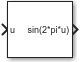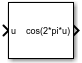# Sine 和 Cosine 模块

• 库：

••## 说明

Sine 和 Cosine 模块通过利用象限波对称性的查找表方法来实现定点正弦和/或余弦波。模块可以输出输入信号的以下函数，具体取决于您为 Output formula 参数选择的设置：

• sin(2πu)

• cos(2πu)

• exp(j2πu)

• sin(2πu) and cos(2πu)

## 端口

### 输出

#### 依存关系

Output formula 设置为 “`sin(2*pi*u)`” 或 “`sin(2*pi*u) and cos(2*pi*u)`” 时，此端口启用。

#### 依存关系

Output formula 设置为 “`cos(2*pi*u)`” 或 “`sin(2*pi*u) and cos(2*pi*u)`” 时，此端口启用。

`exp(j*2*pi*u)`”，使用查找表方法实现。

#### 依存关系

Output formula 设置为 “`exp(j*2*pi*u)`” 时，此端口启用。

## 参数

#### 编程用法

 模块参数：`Formula` 值：`'sin(2*pi*u)' | 'cos(2*pi*u)' | 'exp(j*2*pi*u)' | 'sin(2*pi*u) and cos(2*pi*u)'`

#### 编程用法

 模块参数：`NumDataPoints` 类型：标量 值：`integer >= 2` 默认值：`'(2^5)+1'`

#### 编程用法

 模块参数：`OutputWordLength` 类型：标量 值：`integer from 2 to 53` 默认值：`'16'`

#### 编程用法

 模块参数：`InternalRulePriority` 值：`'Speed' | 'Precision'` 默认值：`'Speed'`

## 模块特性

 数据类型 `Boolean` | `double` | `fixed point` | `integer` | `single` 直接馈通 `否` 多维信号 `否` 可变大小信号 `否` 过零检测 `否`

## 另请参阅

| | (HDL Coder) | (HDL Coder)